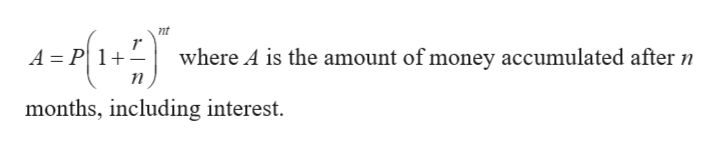# Use the present value formula to determine the amount to be invested​ now, or the present value needed. The desired accumulated amount is\$120,000 after7 years invested in an account with4% interest compoundedquarterly.Whats the amount to be invested now or the present value needed? (round to the nearest cent)

Question
6 views
Use the present value formula to determine the amount to be invested​ now, or the present value needed.

The desired accumulated amount is\$120,000 after
7 years invested in an account with
4% interest compounded
quarterly.
Whats the amount to be invested now or the present value needed? (round to the nearest cent)
check_circle

Step 1

Given information:

Accumulated amount (A)= \$120,000

Rate of interest (r) = 4% = 0.04

Number of years (t) = 7

Compounded quarterly (n) = 4

Step 2

Compound interest...help_outlineImage Transcriptionclosent A-H2) A P 1+ where A is the amount of money accumulated after n months, including interest fullscreen

### Want to see the full answer?

See Solution

#### Want to see this answer and more?

Solutions are written by subject experts who are available 24/7. Questions are typically answered within 1 hour.*

See Solution
*Response times may vary by subject and question.
Tagged in

### Math